Disclaimer: This is an example of a student written assignment.

Any opinions, findings, conclusions or recommendations expressed in this material are those of the authors and do not necessarily reflect the views of UKEssays.com.

# Analysis of Time Slicing Algorithms for Round Robin

 ✓ Paper Type: Free Assignment ✓ Study Level: University / Undergraduate ✓ Wordcount: 5502 words ✓ Published: 10th Nov 2021

## Abstract

Round Robin scheduling algorithm is one of the most common scheduling algorithm used in the real time environment. Its efficiency mainly depends on a Time Quantum which is a set time assigned by the CPU to all the tasks. It is highly critical cause if we choose a lower time quantum value then, the context switch is high and if we choose a higher time quantum then the algorithm behaves like a First-Come-First-Serve (FCFS) manner. So, the performance of the system solely tends to depend majorly on the optimal time quantum. In this paper, we have surveyed different methods to pick the optimal time quantum dynamically and check the performance of each algorithm based on the turn around time,waiting time and response time.

Keywords : Round Robin, Time Quantum, Turn Around Time, Waiting Time, Response Time.

## I. INTRODUCTION

CPU scheduling is one of the fundamental features that an Operating System (OS) needs to perform multitasking. CPU scheduling means allocation of sufficient resources to a process being executed.

A scheduler is what carries out the scheduling activity. Schedulers are often implemented so they keep all computer resources busy (as in load balancing), allow multiple users to share system resources effectively, or to achieve a target quality of service. Scheduling is fundamental to computation itself, and an intrinsic part of the execution model of a computer system; the concept of scheduling makes it possible to have computer multitasking with a single central processing unit (CPU). Several CPU scheduling algorithms have been implemented to meet various OSs requirements, such as Round Robin (RR), Shortest Job First (SJF), Priority, First Come First Served (FCFS), and Shortest Remaining Time First (SRTF). In this work, we focus on RR, which is one of the most widely used algorithm among others.

If you need assistance with writing your assignment, our professional assignment writing service is here to help!

Round Robin Scheduling is a preemptive algorithm which allocates a small amount of time called time quantum (TQ) for each ready process waiting for execution, equally and in circular fashion, treating all processes fairly without priority. It is highly preferred in time-sharing and real-time OSs. In spite of its advantages, it suffers low throughput (the number of processes that are completed per unit of time), high turnaround time (the time between starting and completion), high waiting time (the amount of time that is waiting in the ready queue), and large number of context switches. The most interesting issue with the Round Robin algorithm is the time quantum.

a. Setting a small TQ causes too many context switches which results in a low performance of the CPU .

b. Setting the TQ to a large value may cause a poor response time and the RR algorithm tends to work as FCFS.

Different CPU scheduling algorithms have different properties and the choice of a particular algorithm May favors one class of processes over another. In choosing which algorithm to use in a particular situation, we must consider the properties of the various algorithms.

CPU Utilization: We want to keep the CPU as busy as possible. Conceptually, CPU utilization can range from 0 to 100 percent. In a real system, it should range from 40 percent (for a lightly loaded system) to 90 percent (for a heavily used system) .

Throughput: If the CPU is busy executing processes, then work is being done. One measure of work is the number of processes that are completed per time unit, called throughput. For long processes, this rate may be one process per hour; for short transactions, it may be 10 processes per second .

Turnaround time: From the point of view of a particular process, the important criterion is how long it takes to execute that process. The interval from the time of submission of a process to the time of completion is the turnaround time .

Waiting time: The CPU scheduling algorithm does not affect the amount of time during which a process executes or does I/O; it affects only the amount of time that a process spends waiting in the ready queue .

Response time: In an interactive system, turnaround time may not be the best criterion. Often, a process can produce some output fairly early and can continue computing new results while previous results are being output to the user. Thus, another measure is the time from the submission of a request until the first response is produced .

## II. LITERATURE REVIEW OF VARIOUS ROUND ROUND ALGORITHM BASED ON THE OPTIMAL TIME QUANTUM SELECTION

### Assumption

For analysis purposes we have considered only CPU bound processes. In each test case 5 independent processes are analysed in a single process environment where the burst time and arrival time are known before execution. The table below illustrates the same.

 Process AT BT P1 0 45 P2 6 90 P3 10 20 P4 18 35 P5 20 80

We use the following formulas to calculate the turn around time (TAT) and waiting time (WT).

TAT = COMPLETION TIME - ARRIVAL TIME

WT = TAT - BURST TIME

### Modified Round Robin Algorithm for Resource Allocation in Cloud Computing 

This algorithm starts with the time quantum being equal to the burst time of first task coming in, which changes after the completion of the first request. When a new task is added into the ready queue, the algorithm calculates the average of sum of the burst time of tasks found in the ready queue including the new arrived task.

The Gantt Chart for the case considered is shown below: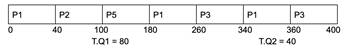The turn around time , waiting time and response time is calculated in the below table.

 Process TAT WT P1 45 0 P2 264 174 P3 55 35 P4 82 47 P5 214 134

### Self-Adjustment Time Quantum in Round Robin Algorithm Depending on Burst Time of the Now Running Processes 

In this algorithm, the optimal time quantum is calculated by finding the median for the set of processes in the ready queue which are first sorted in ascending order of their burst time.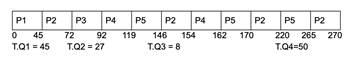The Gantt Chart for the case considered is shown below:

The turn around time, waiting time and response time is calculated in the below table.

 Process TAT WT RT P1 45 0 0 P2 264 174 39 P3 82 62 62 P4 144 109 74 P5 245 165 99

### Implementation of Alternating Median Based Round Robin Scheduling Algorithm 

The proposed algorithm here uses a set of two time quanta for scheduling the tasks.Before the first cycle of scheduling begins, the first time quanta is set equal to the median of burst time of processes present in the ready queue while the second one is calculated as the difference between the highest value of burst times and the first time quanta. These time quanta remain fixed throughout scheduling and are not recalculated until the architecture of the ready queue gets changed by the arrival of a new process. The larger time quantum is used if an even number of cycles of scheduling are completed while the smaller time quantum is used if a odd number of cycles of scheduling are completed.

The Gantt Chart for the case considered is show below :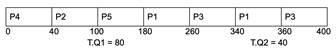The turn around time, waiting time and response time is calculated in the table below :

 Process TAT WT P1 360 260 P2 100 40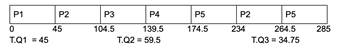Process TAT WT P3 400 280 P4 40 0 P5 180 100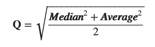### Modulus based Round Robin scheduling algorithmThe Modulus Based (MB) algorithm has been built on the basis of two scheduling algorithms namely AN algorithm and Shortest Remaining Burst Round Robin algorithm.This algorithm calculates the time quantum per cycle due to change in execution time of tasks that occur in every cycle. The formula of calculation of time quantum Q is given in equation below :

The Gantt Chart for the case considered is show below:

The turn around time , waiting time and response time is calculated in the table below:

 Process TAT WT RT P1 45 0 0 P2 264 174 151 P3 55 35 35 P4 82 47 47 P5 217 137 80

### An improved dynamic Round Robin scheduling algorithm based on a variant quantum time 

In this algorithm we first sort all the processors in the ready queue based on their burst time.If the arrival time of all process is same then time quantum is calculated using below formula:

T.Q1=Σ of two high B.T/2.

T.Q2=Σ of high remaining B.T and T.Q1/2.

Assign T.Q1 to first cycle and for remaining cycle use

T.Q2.If the arrival time of all process is different than time quantum is calculated using below formula.

T.Q1=B.T of first arrived process

T.Q2= (Σ of high remaining B.T and T.Q1 /2) – (Σ of the two lowest remaining AT/2 (used only once time)

T.Q3= (Σ of high remaining B.T and T.Q1 /2) – (lowest remaining AT/2)

Assign this time quantum T.Q1 to first cycle and for second cycle used T.Q2 and for remaining cycle used T.Q3.

The Gantt Chart for the case considered is show below:

The turn around time,waiting time and response time is calculated in the table below:

 Process TAT WT RT P1 45 0 0 P2 174.5 84.5 39 P3 129.5 109.5 94.5 P4 156.5 121.5 121.5 P5 265 185 154.5

### Adaptive Round Robin Scheduling using Shortest Burst Approach Based on Smart Time Slice 

In this algorithm, the user is to assign priority to the system based on execution time or burst time. The time slice will be based on all CPU burst of new running processes. The smart time slice calculated according to the processes burst time; number of processes present in the ready queue are odd : smart time slice = mid process burst time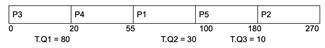The number of processes present in the ready queue are even:

smart time slice = average of all processes burst time is assigned to the processes.

The Gantt Chart for the case considered is show below:

The turn around time,waiting time and response time is calculated in the table below:

 Process WT TAT RT P1 55 100 55 P2 180 264 174 P3 0 10 0 P4 20 37 2 P5 100 160 80

### Best Time Quantum RR CPU Scheduling Algorithm []

In this algorithm, the number of processes is residing in the ready queue, we assume their arrival time is assigned to zero and burst times are allocated to the CPU. The burst time and the number of processes (n) are accepted as input. Now first of all we arrange all processes in increasing order according to their given burst time and choose best time slice with the help of mean and median. The best time slice will depend on the mean and median of burst time.

The Gantt Chart for the case considered is show below:

The turn around time, waiting time and response time is calculated in the table below:

 Process TAT WT RT P1 45 0 0 P2 264 174 174 P3 55 35 35 P4 82 47 47 P5 160 80 80

### Eighty-Five Percentile Round-Robin algorithm (EFPRR) 

The proposed algorithm further improves the other previously published RR based algorithms via calculating the TQ at the beginning of each step using the 85 percentile of the processes' burst time. After arranging the processes in an increasing order based on their CPU time, the TQ is computed via multiplying the mean of all processes' time with the 85 percentile constant (85%*mean).

Then, it checks the remaining time of the process. If it is lesser than or equal to the calculated TQ, the processor executes the running process until completion. Or else, the process will be send to the tail of the ready queue.

The turn around time , waiting time and response time is calculated in the table below: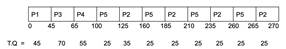Process TAT WT RT P1 100 55 55 P2 269 179 159 P3 20 0 0 P4 37 2 2 P5 165 80 80

### Optimize task scheduling act by modified round robin scheme with vigorous time quantum

The proposed approach do a sorting procedure in ready queue to arrange existing process in increasing order on the base of their burst time. Typically, calculated time slice i.e. average burst time is a time quantum period for each process, each and every process executes over CPU with set time period.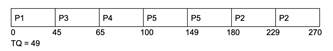If process completely executed before set time period then approach check ready queue to confirm that no new process has occur in ready. If new process occurs then again sorting process has executed and followed that the designed approach recalculates the new time quantum for further process. In case when no new process arrives in ready queue the approach skip sorting procedure but recalculate the new effective time quantum on the base of remaining procedure of ready queue.

Our academic experts are ready and waiting to assist with any writing project you may have. From simple essay plans, through to full dissertations, you can guarantee we have a service perfectly matched to your needs.

At the condition when executed process not completely finished within set quantum time then after execution approach has verify that required burst time of current executed process is less than from current set time quantum. If remaining burst time of current executed process less than from quantum time than designed approach again execute same process without switching the process in memory else the current process will take last place in queue and wait too for turn. Additionally at this phase proposed approach again performs same operation from sorting procedure. The whole procedure will execute in cycle form until task of system not completed.

 Process TAT WT Response time P1 45 0 0 P2 264 174 174 P3 55 35 35 P4 82 47 47 P5 160 80 80

## III. RESULTS

### An Effective Round Robin Algorithm using Min-Max Dispersion Measure

The proposed algorithm further improves the minmax algorithm by sorting the processes in the ready queue in a non-decreasing order. The time quantum is then calculated by subtracting the minimum burst time from the maximum burst time of the processes in the ready queue. If this difference is less than 25, the time quantum is set to 25. This is repeated every time a new process enters the ready queue.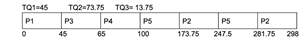The turn around time , waiting time and response time is calculated in the table below:

 Process TAT WT RT P1 45 0 0 P2 264 174 119 P3 55 35 35 P4 82 47 47 P5 245 165 80

### Improved Round Robin Scheduling using Dynamic Time Quantum (IRR ALGORITHM)

 Process TAT WT RT P1 45 0 0 P2 292 202 39 P3 55 35 62 P4 82 47 74 P5 261.75 181.75 99
 Average Waiting Time Average Turn Around Time Average Response Time AVGBT 78 132 62 SRBRR 102 156 54.8 MODULUS 78 132 62 DRR 100.1 154.1 81 STS 71 114.2 62 MRR 67.2 121 67 85PRR 124 118 59 RRLONG 94.2 137.4 111 MRRSVTQ 120 200 70 MINMAX 84.2 138.2 56.2

Then time quantum is calculated using below formula

T.Q= ( MEDIAN + HIGH B.T ) / 2

Step-1:process arrive in ready queue based on A.T.

Step-2:sort the process which found in ready queue. Step-3:using above formula find time quantum of B.T of process which is placed on ready queue.

Step-4:now assign this time quantum to all process which is loaded in ready queue.

Step-5:if process B.T-time quantum=0 then terminate that process.

Step-6:IF process B.T-TIME QUANTUM!=0 then put that process at end of ready queue.Continue with step:2 to step:7 until all process completed.

Step-7:calculate T.A.T,W.T,NO OF C.S.

## IV. CONCLUSION

Using a common input we calculated the average turnaround time, average waiting time and response time for each of the above algorithms and displayed the results we got in the form of a bar graph.

Based on the inputs we used, we found that the 85PRR algorithm has the best average turnaround time and average wait-time.

The SRBRR algorithm has the best response time.

## References

1. Modern Operating Systems (4th Edition) by Andrew S. Tanenbaum, 2008 by Pearson Education, Inc., Upper Saddle River, New Jersey, 07458, Pearson Prentice-Hall.

2. Anju Muraleedharan, Neenu Antony, R. Nandakumar, "Dynamic Time Slice Round Robin Scheduling Algorithm with Unknown Burst Time", Indian Journal of Science and Technology, Vol 9(8),pp.1- 6, February 2016.

3. Imran Qureshi, "CPU Scheduling Algorithms: A Survey", Int. J. Advanced Networking and Applications, Vol 5(4), pp.1968-1973 (2014).

4. Ajit Singh, Priyanka Goyal, Sahil Batra," An Optimized Round Robin Scheduling Algorithm for CPU Scheduling", (IJCSE) International Journal on Computer Science and Engineering Vol. 02, No. 07, 2383-2385, 2010.

5. Y. H. Jbara, "A new Improved Round Robin-Based Scheduling Algorithm-A comparative Analysis," 2019 International Conference on Computer and Information Sciences (ICCIS), Sakaka, Saudi Arabia, 2019, pp. 1-6. doi: 10.1109/ICCISci.2019.8716476

6. Prafulla Ku. Behera, Pandaba Pradhan and B N B Ray, "Modified Round Robin Algorithm for Resource Allocation in Cloud Computing ", ScienceDirect, Procedia Computer Science 85 ( 2016 ) 878 – 890,2016.

7. Rami J. Matarneh,"Self-Adjustment Time Quantum in Round Robin Algorithm Depending on Burst Time of Now Running Processes", American J. of Applied Sciences 6(10): 1831 - 1837,2009

8. Ali Javed , Naveed Ghaffar and Salman Arif "Implementation of Alternating Median Based Round Robin Scheduling Algorithm",IEEE, International Conference on Computer and Information Technology,2016.

9. Badal Dave, Surendra Yadev, Manish Mathuria, Yatendra Mohan Sharma, "Optimize task scheduling act by modified round robin scheme with vigorous time quantum",IEEE,International Conference on Intelligent Sustainable Systems (ICISS),2017.

10. Saad Rehman,Farhan Riaz and Salman Arif, "Design of A Modulus Based Round Robin Scheduling Algorithm",IEEE, 9th Malaysian Software Engineering Conference, Dec. 2015

11. A. Alsheikhy, R. Ammar, and R. Elfouly, "An improved dynamic Round Robin scheduling algorithm based on a variant quantum time",IEEE, 2015 11th International Computer Engineering Conference (ICENCO), 2015, pp. 98-104.

12. Sanjaya Kumar Panda, Sourav Kumar Bhoi,"An Effective Round Robin Algorithm using Min-Max Dispersion Measur",IJCSE, International Journal on Computer Science and Engineering (IJCSE), 2012.

13. Saroj Hiranwal,Dr. K.C.Roy, "Adaptive Round Robin Scheduling using Shortest Burst Approach Based on Smart Time Slice", International Journal of Data Engineering (IJDE), Volume 2, Issue 3

View all

## DMCA / Removal Request

If you are the original writer of this assignment and no longer wish to have your work published on UKEssays.com then please:

Related Services

Prices from

S\$203

Approximate costs for:

• 1000 words
• 7 day deliveryHumanity University

Dedicated to your worth and value as a human being!

Related Lectures